### Home > MC1 > Chapter 9 > Lesson 9.2.1 > Problem9-59

9-59.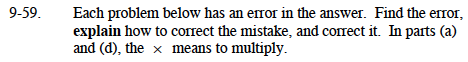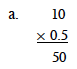Where should the decimal point be?
Does 50 make sense as an answer?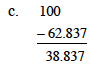Here is a similar problem: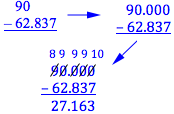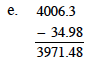3971.32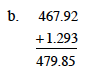Make sure that like parts are being added (tenths to tenths, hundredths to hundredths, etc.).
Lining up the decimal points can help to keep track of like parts.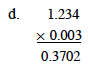Thinking about the denominators of the fractions can help make sense of where the decimal point should be.

$\text{What happens if you multiply }1\frac{234}{1000}\text{ by }\frac{3}{1000}?$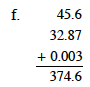Make sure that you add like parts.
Remember that lining up the decimal points can help to keep track of like parts.